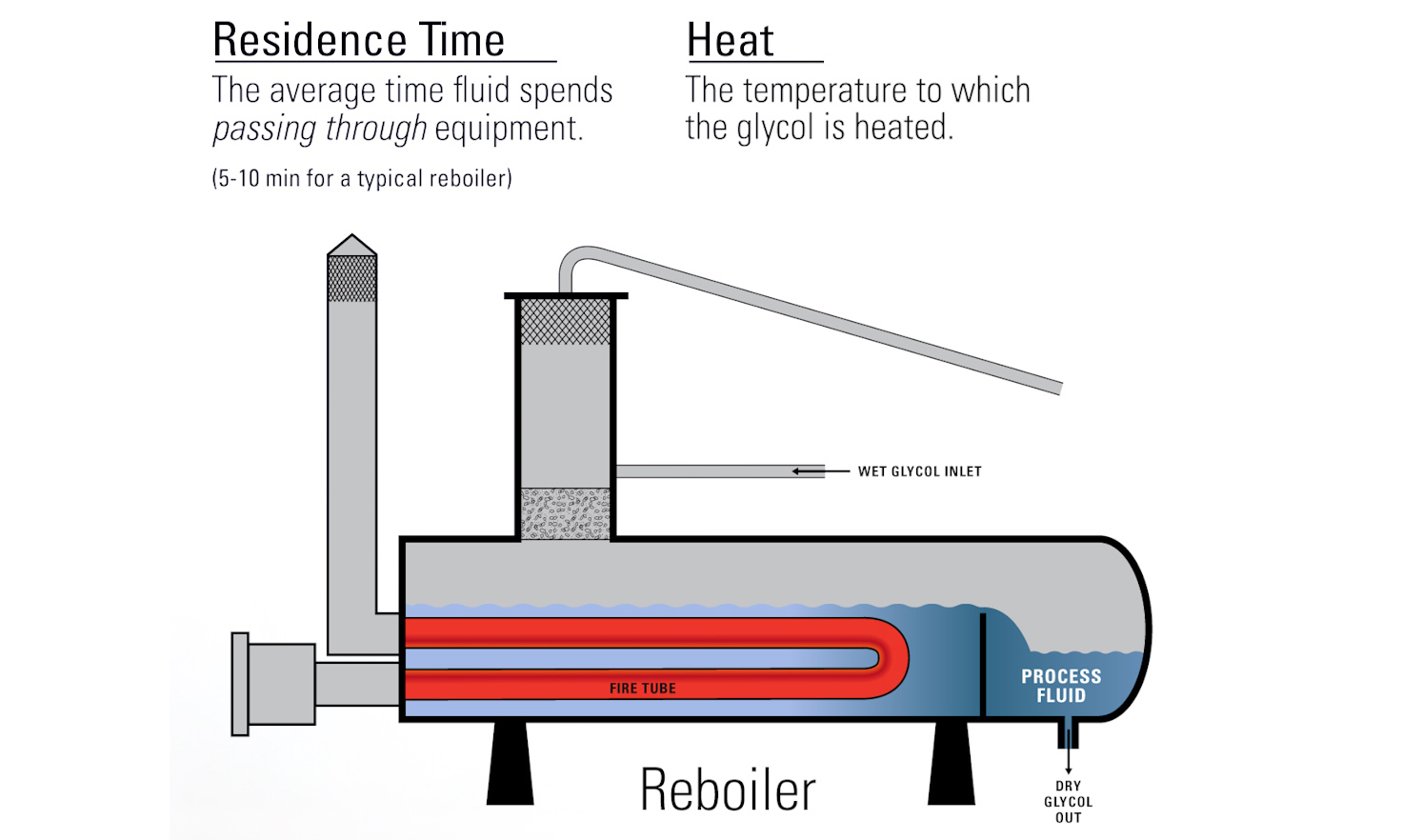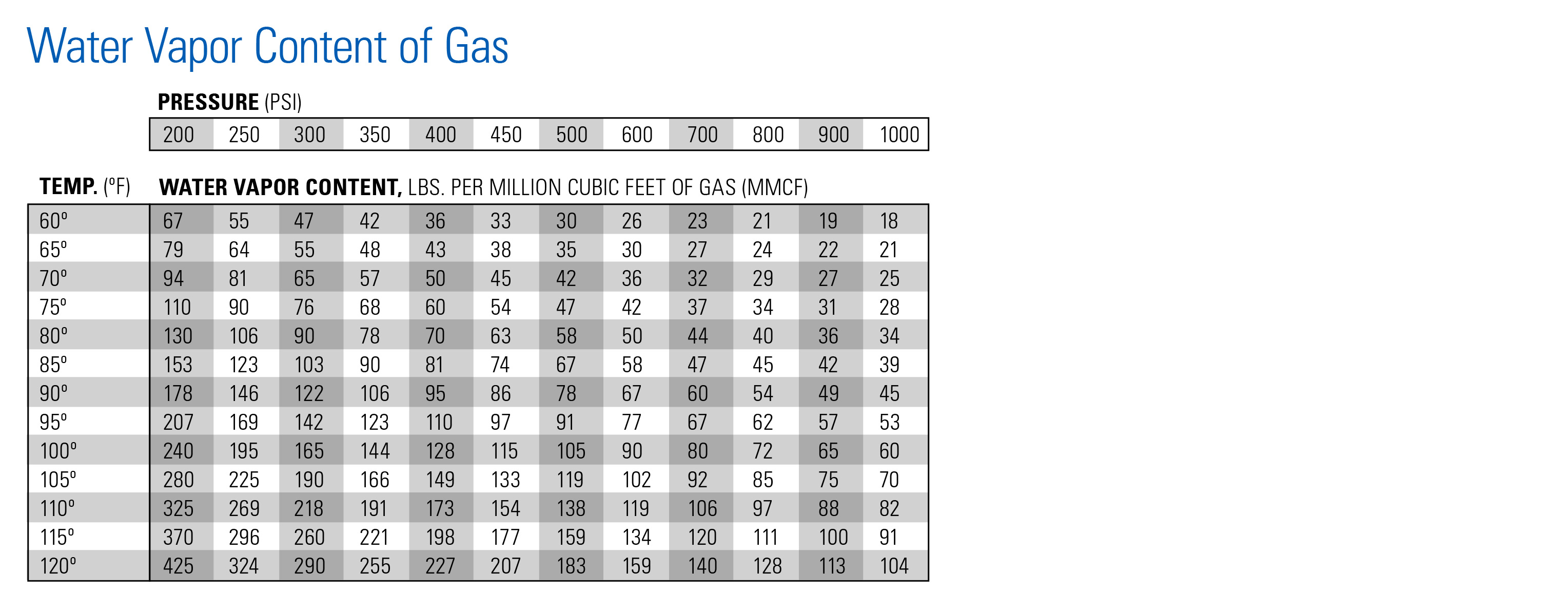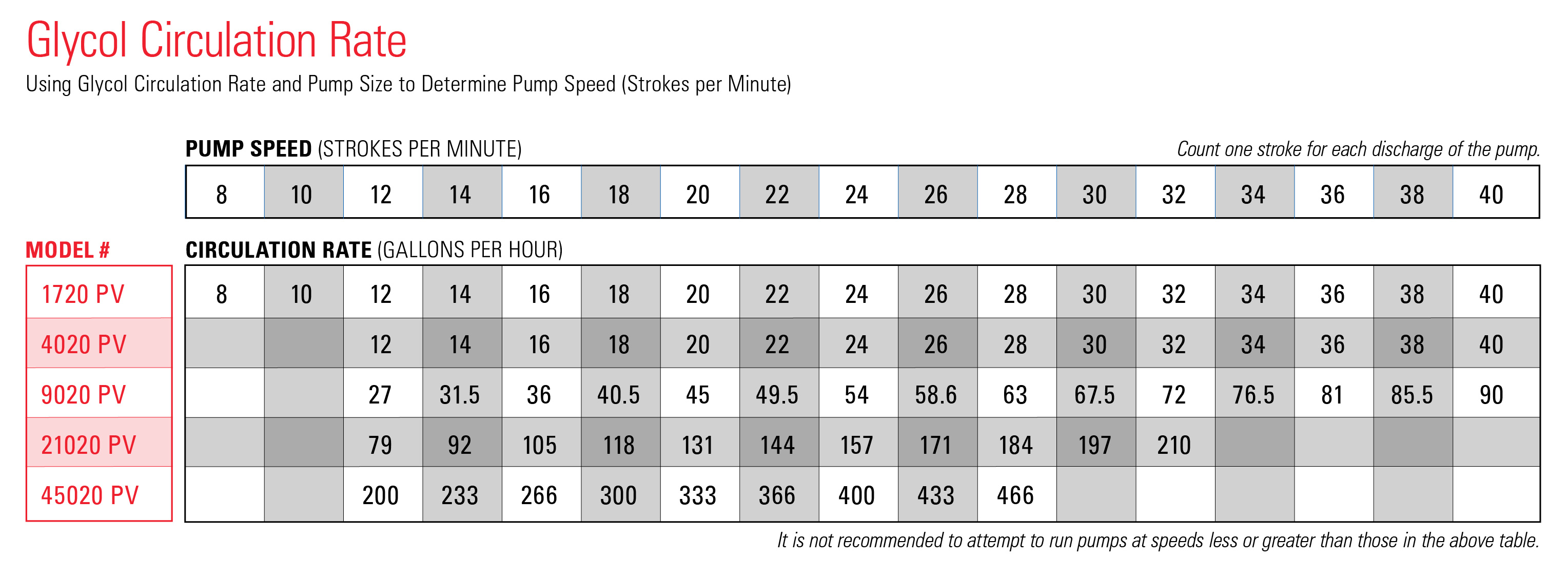# TEG Circulation Rate CalculationNatural gas dehydration is a process gas producers use to remove water vapor from their gas flow stream.

To do this, producers introduce triethylene glycol into their gas.

In this blog, we’ll show you how to calculate the amount of triethylene glycol (TEG) you need to run through your dehydration system to meet dew point, and help you size the right glycol pump to circulate the TEG.

## Residence time & Heat in glycol circulation

Generally, a glycol circulation rate of 3-5 gallons per pound of water to be removed is sufficient to adequately dehydrate the gas. However, if the glycol flow rate is too high, it can overwhelm the reboiler and you will lose efficiency.

There are two factors that help to separate the water from the glycol: Residence time and heat.

If you don’t allow the glycol enough time to reside in the reboiler, it will not increase the temperature of the glycol enough to remove the water and achieve optimal purity level, which is 99% or more.

If the glycol flow rate is too low, you will not be removing enough water vapor from your gas traveling through the contact tower to achieve your desired dew point level.## The TEG Circulation rate calculation

Here’s how to calculate your glycol circulation rate to determine your glycol pump speed. We've also created a PDF guide that you can download below that walks you through these same steps.

### Step 1:

Use the Water Vapor Content of Gas Chart to Identify your water content (w).

• Let’s use an example of 80-degree gas at 600 psi. That tells us the water content is 50 lbs. per million cubic feet of gas.This chart shows the water content of your natural gas at various pressures and temperatures. This is the maximum amount of water you can expect your natural gas production to hold at the given pressures and temperatures.

### Step 2:

Multiply your water content (w) by the gas flow rate (m).

• In this example, our flow rate is 4 million. So, we multiply 50 (w) by 4 (m).
• This gives us 200 lbs. of total water to be removed per day

### Step 3:

Multiply total water content—200—by gallons of glycol needed to remove one pound of water (g), which is 3-5 depending on glycol purity and temperature, so in our example we’ll use 4.

• This gives us 800 gallons of glycol per day.

### Step 4:

Divide total amount of circulated glycol per day by 24 to calculate the per-hour rate.

• 800 divided by 24 gives us 33.3 gallons per hour circulation.

### Step 5:

Using the Glycol Circulation Rate Chart, identify your Kimray pump size and find the circulation rate closest to your calculated rate to determine the ideal pump speed.

• In this example, we’re using a 9020PV which puts our 33.33 between 14 and 16 strokes per minute.
• As a general rule, round up to the nearest stroke count, which is 16 strokes per minute (SPM). This is the pump speed we need to maintain your circulation rate of 800 gallons per day.This chart will help us size the correct glycol pump, and calculate the number of strokes our pump needs to make.

Now that you have this number, you can change your pump speed to match your conditions and dry your gas sufficiently. To do this, adjust your needle valves equally, counting the strokes per minute to verify the correct change has been made.

Your glycol flow rate should be checked on a regular basis to prevent both over-circulation and under-circulation.